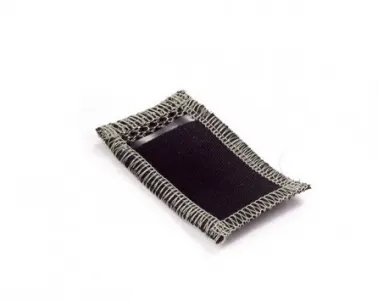Project tutorial# Analog Textile Press Button Tutorial © GPL3+

How to read the value of a Analog textile press button with your Arduino.

• 2,054 views
• 7 respects

## Components and supplies

### 1. Introduction

The Analog textile pressbutton is a analog textile sensor that changes its resistance if you put pressure on it. It is useful for creating variable voltage divider that can be easily read by an Arduino.

### 2. Connect the sensor to the power supply

Connect one end of the sensor to the 5V pin of the Lilypad. Then you need to connect the other end to ground through a 1 kOhm resistor like shown in the picture.

### 3. Connect the sensor to the Arduino

Now connect the analog input ping A5 of the Arduino to the end of the sensor connected to the resistor.

### 4. How to read values from sensor

The sensor changes its resistance depending on how much you press it. This will change the voltage read by the Arduino. The read value will change the blinking frequency of the LED.

``````int sensorPin = A5;    // select the input pin for the sensor
int ledPin = 13;      // select the pin for the LED
int sensorValue = 0;  // variable to store the value coming from the sensor
void setup() {
// declare the ledPin as an OUTPUT:
pinMode(ledPin, OUTPUT);
}
void loop() {
// read the value from the sensor:
// turn the ledPin on
digitalWrite(ledPin, HIGH);
// stop the program for <sensorValue> milliseconds:
delay(sensorValue);
// turn the ledPin off:
digitalWrite(ledPin, LOW);
// stop the program for for <sensorValue> milliseconds:
delay(sensorValue);
}
``````

## Code

##### Code snippet #1Arduino
```int sensorPin = A5;    // select the input pin for the sensor
int ledPin = 13;      // select the pin for the LED
int sensorValue = 0;  // variable to store the value coming from the sensor

void setup() {
// declare the ledPin as an OUTPUT:
pinMode(ledPin, OUTPUT);
}

void loop() {
// read the value from the sensor:
// turn the ledPin on
digitalWrite(ledPin, HIGH);
// stop the program for <sensorValue> milliseconds:
delay(sensorValue);
// turn the ledPin off:
digitalWrite(ledPin, LOW);
// stop the program for for <sensorValue> milliseconds:
delay(sensorValue);
}
```

July 8, 2016

#### Members who respect this project

See similar projects
you might like

• 2,943 views
• 6 respects

#### Soft potentiometer tutorial

Project tutorial by Arduino_Scuola

• 2,053 views
• 0 respects

by RoHa

• 5,651 views
• 13 respects

#### A Cool Scale

Project tutorial by Arduino_Scuola

• 4,046 views
• 1 comment
• 1 respect

#### Slack Away Button with Wia Dot One and Button Module

Project tutorial by Team Wia

• 1,599 views